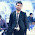### C++ program to solve the equation of 2 variables

/*C++ program to solve the equation of 2 variables
by Mohit Rajput */

#include
#include
class equation
{
private:
float a,b,c;
float x,y;
public:
void get()
{
cout<<"\nEnter coefficients a,b & c:";
cin>>a>>b>>c;
}

void printf(equation w)
{
cout<<"\n"<
}

void calc(equation p,equation q)
{
y=(p.c-q.c)/((p.b*q.a)-(q.b*p.a));
x=(p.c-p.b*y)/p.a;
}

void result()
{
cout<<"\nx="<
cout<<"\ny="<
}

};

void main()
{
clrscr();
equation p,q,r;
cout<<"\nFor 1st equation\n";
p.get();
cout<<"\nFor 2nd equation\n";
q.get();
r.calc(p,q);
cout<<"\nSolution is:\n";
r.result();
getch();
}

1.